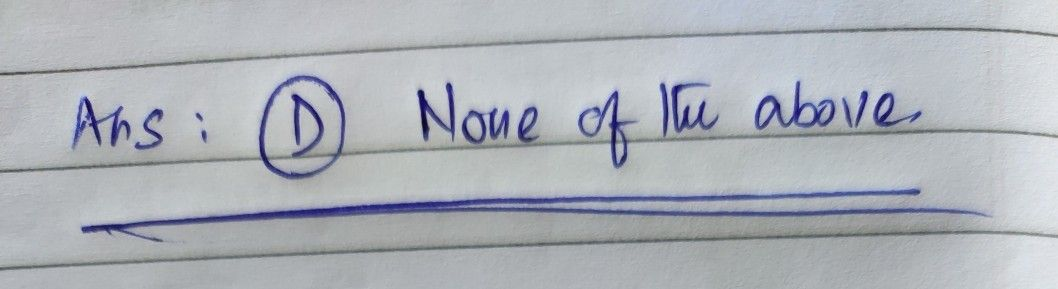Symbol
Problem$Q5$ Linear differential equation is $Oo53$ $A$ $-A$ A differential equation in which all derivatives are in first order $B$ $-A$ A differential equation in which the dependent variable and their derivatives are in first degree $C$ $A$ A differential equation in which the dependent variable and their derivatives are in first degree and are $-$ multipled $D$ $→$ O None of the above $2$
Calculus
SolutionQanda teacher - pragya